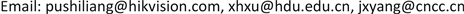﻿ 无线传感器网络中实现可信信息覆盖的能量感知的节点优化选择方法研究 Research on Energy-Aware Sensor Selection for Confidential Information Coverage in Wireless Sensor Networks

Computer Science and Application
Vol.07 No.12(2017), Article ID:23074,7 pages
10.12677/CSA.2017.712135

Research on Energy-Aware Sensor Selection for Confidential Information Coverage in Wireless Sensor Networks

Shiliang Pu1, Xianghua Xu2, Jianxu Yang3

1Hangzhou Hikvision Digital Technology Co., Ltd., Hangzhou Zhejiang

2School of Computer Science, Hangzhou Dianzi University, Hangzhou Zhejiang

3China National Bank Clearing Center, BeijingReceived: Dec. 1st, 2017; accepted: Dec. 13th, 2017; published: Dec. 20th, 2017ABSTRACT

In the area monitoring applications of wireless sensor networks, the optimal selection of sensor nodes for confidential information coverage is an important issue. This paper presents an energy aware method of sensor node selection to satisfy the monitoring precision and sensor residual energy constraint. We transform the energy aware sensor node selection problem into a 0-1 integer programming problem, and solving the minimum selected sensor set, both to ensure the accuracy of monitoring and prolong the network lifetime. We use Intel Berkeley laboratory’s temperature data for simulation experiments. Compared with different interpolation methods, the network lifetime is extended by more than 2 times under the same monitoring precision error condition.

Keywords:WSN, Sensor Deployment, Network Lifetime, Sensor Node Selection, Energy Aware, 0-1 Integer Programming

1杭州海康威视数字技术股份有限公司，浙江 杭州

2杭州电子科技大学计算机学院，浙江 杭州

3中国人民银行清算总中心，北京1. 引言

Wang B  针对满足可信信息覆盖的节点选择问题，利用Kriging插值法预估未部署点的监测值，以及用均方根误差对结果进行分析评价，但是并没有论证搜索半径对结果精度的影响。在   中，S. P. Chepuri等人将传感器选择问题解释为一种优化问题，即在网络中满足误差约束的条件下选择最佳的传感器子集，设计了一种稀疏选择向量选择最优传感器集合。文献   虽然考虑了误差精度，将传感器选择问题转化为0-1整数规划问题求解；然而S. P. Chepuri等人并有没考虑所选传感器节点的剩余能量因素，若所选传感器的剩余能量处于低能量，那么被选择的节点将很快失效。在  中，Sijia Liu等人针对传感器选择问题设计了一种稀疏促进罚函数避免重复选择相同传感器的情况，将问题公式化为凸二次规划求解，从而达到网络中传感器负载均衡的目的。然而同样作为区域重构问题，Sijia Liu等人将估计的误差精度作为凸二次规划的目标函数，这就使得产生的传感器集合存在并没有达到给定精度要求的情况。

 在可信信息覆盖的区域监测传感器选择问题中引入剩余能量约束，避免选择剩余能量过低的传感器，延长网络寿命并保证区域监测覆盖和监测值精度。

 提出了能量约束的传感器选择问题的优化求解方法，将传感器优化选择问题转化为0-1整数规划问题，并求解最少传感器选择集合，实现可信信息覆盖。

 利用Inter Berkeley实验室的温度数据进行仿真实验，并针对不同的插值方法进行了实验对比分析，在满足相同监测精度误差情况下，网络寿命延长了2倍以上。

2. 问题定义

${y}_{k,n}=f\left({t}_{k},{s}_{n}\right)+{e}_{k,n}$ (1)

$y={\left[{y}_{1,1},\cdots ,{y}_{K,1},\cdots ,{y}_{1,N},\cdots ,{y}_{K,N}\right]}^{\text{T}}$

$f=\left[f\left({t}_{1},{s}_{1}\right),\cdots ,f\left({t}_{K},{s}_{1}\right),\cdots ,f\left({t}_{1},{s}_{N}\right),\cdots ,f\left({t}_{K},{s}_{N}\right)\right]$

$e={\left[{e}_{1,1},\cdots ,{e}_{K,1},\cdots ,{e}_{1,N},\cdots ,{e}_{K,N}\right]}^{\text{T}}$

$y=f+e$ (2)

$\theta =\left\{f\left({t}_{1},\delta \right),f\left({t}_{2},\delta \right),\cdots ,f\left({t}_{k},\delta \right)\right\}$ (3)

$\stackrel{¯}{\theta }=WF\left(y\right)+c$ (4)

$\stackrel{¯}{\theta }=Wy$ (5)

$J\left(w\right)={\sum }_{n=1}^{N}E{\left[{\theta }_{n}-{\left({w}_{n}\right)}^{\text{T}}F\left(y\right)\right]}^{2}$ (6)

$J\left(w\right)={\sum }_{n=1}^{N}E\left[{\theta }_{n}^{2}-2{w}_{n}^{\text{T}}F\left(y\right)\theta +{w}_{n}F\left(y\right)F{\left(y\right)}^{\text{T}}{w}_{n}^{\text{T}}\right]$ (7)

$E\left(F\left(y\right)F{\left(y\right)}^{\text{T}}\right)=E\left[F\left(f+e\right)F{\left(f+e\right)}^{\text{T}}\right]=p$

$E\left(F\left(y\right)\theta \right)=E\left[F\left(f+e\right)\theta \right]=E\left[F\left(f\right)\ast f\left({t}_{k},\delta \right)\right]=q$

$E\left({\theta }_{n}^{2}\right)=N{\sigma }_{f}^{2}$

$J\left(w\right)=N{\sigma }_{f}^{2}-2{\sum }_{n=1}^{N}q{w}_{n}+{\sum }_{n=1}^{N}{\left({w}_{n}\right)}^{\text{T}}p{w}_{n}$ (8)

${w}_{n}{\lambda }_{i}\ge \lambda ,\text{\hspace{0.17em}}n=1,\cdots ,n$ (9)

$\begin{array}{l}\text{minimize}{‖{w}_{n}‖}_{0,1}\\ \left\{\begin{array}{l}J\left(w\right)={\sum }_{n=1}^{N}{\left({w}_{n}\right)}^{\text{T}}p{w}_{n}-2{\sum }_{n=1}^{N}q{w}_{n}\le ϵ\\ {w}_{n}{\lambda }_{i}\ge \lambda ,\text{\hspace{0.17em}}n=1,\cdots ,n\\ ‖{w}_{n}‖\ge 1\end{array}\end{array}$ (10)

3. 仿真实验Figure 1. The error’s effect on the life cycle of the networkTable 2. Nodes selection with different thresholdsFigure 2. The network lifetime of our node selection algorithm is compared with algorithm  that does not consider the residual energy

4. 总结

Research on Energy-Aware Sensor Selection for Confidential Information Coverage in Wireless Sensor Networks[J]. 计算机科学与应用, 2017, 07(12): 1199-1205. http://dx.doi.org/10.12677/CSA.2017.712135

1. 1. Liu, S., Chepuri, S.P., Fardad, M., et al. (2016) Sensor Selection for Estimation with Correlated Measurement Noise. IEEE Transactions on Signal Processing, 64, 3509-3522. https://doi.org/10.1109/TSP.2016.2550005

2. 2. Kail, G., Chepuri, S.P. and Leus, G. (2017) Robust Censoring Using Metropolis-Hastings Sampling. IEEE Journal of Selected Topics in Signal Processing, 10, 270-283. https://doi.org/10.1109/JSTSP.2015.2506142

3. 3. Wang, B., Deng, X., Liu, W., et al. (2013) Confident Information Coverage in Sensor Networks for Field Reconstruction. Wireless Communications IEEE, 20, 74-81. https://doi.org/10.1109/MWC.2013.6704477

4. 4. Chepuri, S.P. and Leus, G. (2015) Continuous Sensor Placement. Signal Processing Letters IEEE, 22, 544-548. https://doi.org/10.1109/LSP.2014.2363731

5. 5. Chepuri, S.P. and Leus, G. (2014) Sparsity-Promoting Adaptive Sensor Selection for Non-Linear Filtering. Proceedings of IEEE International Conference of Acoustics, Speech and Signal Processing (ICASSP), May 2014, 5100-5104. https://doi.org/10.1109/ICASSP.2014.6854570

6. 6. Liu, S., Masazade, E., Fardad, M., et al. (2014) Sparsity-Aware Field Estimation via Ordinary Kriging. 2014 IEEE International Conference on Acoustics, Speech and Signal Processing (ICASSP), 3948-3952. https://doi.org/10.1109/ICASSP.2014.6854342

7. 7. Jiang, F., Chen, J. and Swindlehurst, A.L. (2013) Linearly Reconfigurable Kalman Filtering for a Vector Process. 2013 IEEE International Conference on Acoustics, Speech and Signal Processing (ICASSP), 5725-5729. https://doi.org/10.1109/ICASSP.2013.6638761

8. 8. Masazade, E., Fardad, M. and Varshney, P.K. (2012) Sparsity-Promoting Extended Kalman Filtering for Target Tracking in Wireless Sensor Networks. Signal Processing Letters IEEE, 19, 845-848. https://doi.org/10.1109/LSP.2012.2220350

9. 9. Chepuri, S.P. and Leus, G. (2013) Sparsity-Promoting Sensor Selection for Non-linear Measurement Models. Processing IEEE Transactions on Signal, 63, 684-698. https://doi.org/10.1109/TSP.2014.2379662

10. 10. Liu, S., Masazade, E. and Varshney, P.K. (2012) Temporally Staggered Sensing for Field Estimation with Quantized Data in Wireless Sensor Networks. IEEE Statistical Signal Processing Workshop (SSP), 512-515. https://doi.org/10.1109/SSP.2012.6319746

11. 11. Liu, S.J., Vempaty, A., Fardad, M., et al. (2014) Energy-Aware Sensor Selection in Field Reconstruction. IEEE Signal Processing Letters, 21, 1476-1480. https://doi.org/10.1109/LSP.2014.2342198

12. 12. Strum, J.F. (1999) Using SeDuMi 1.02, a MATLAB Toolbox for Optimization over Symmetric Cones. Optimization Methods & Sofware, 625-653.

13. 13. Grant, M., Boyd, S. and Ye, Y. (2006) CVX: Matlab Software for Disciplined Convex Programming. Global Optimization, 155-210. https://doi.org/10.1007/0-387-30528-9_7